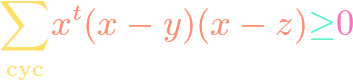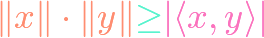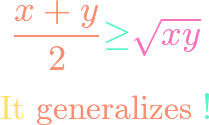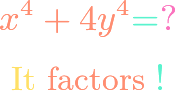# Algebra## Schur’s Inequality

https://www.youtube.com/watch?v=hcK1MoIVbTA In math contest and olympiad circles, Schur’s inequality is known as a result which has special cases that are equivalent to relatively strong results. We prove the ordinary version of Schur’s inequality in this video. Stronger versions have been unearthed in recent years, such as by Valentin Vornicu.## Cauchy-Schwarz Inequality

https://www.youtube.com/watch?v=Oe4Qn_T69-I The real number Cauchy-Schwarz inequality is a multivariable inequality that holds for all real inputs. It has a variety of special cases, including the famous Engel’s form, that have found popular use on math contests and olympiads. Outside of contests, it is useful on optimization problems and in linear algebra. Here, we present an …## Arithmetic-Geometric Mean Inequality

https://www.youtube.com/watch?v=iNldQpfT_ug The arithmetic mean – geometric mean inequailty is a famous multivariable inequality that allows us to compare the sum of some non-negative numbers with their product, in the presence of some other operations. This amazing multivariable inequality has widespread use across mathematics, including on math contests and olympiads. This proof is even more remarkable, …## Sophie Germain Identity

https://www.youtube.com/watch?v=v4YVOMJAuGQ The Sophie Germain identity is a remarkable polynomial factorization. It is frequently useful in math contests, competitions, and olympiads. One would not expect this polynomial to factor, but an ingenious trick via the difference of squares factorization allows for the factorization $$x^4+4y^4 = (x^2-2xy+2y^2)(x^2+2xy+y^2).$$